# Excel INDIRECT Function

Returns a reference to a range

## What is the Excel INDIRECT Function?

The Excel INDIRECT Function returns a reference to a range. The INDIRECT function does not evaluate logical tests or conditions. Also, it will not perform calculations. Basically, this function helps lock the specified cell in a formula. Due to this, we can change a cell reference within a formula without changing the formula itself.

One advantage of this function is that the indirect references won’t change even when new rows or columns are inserted in the worksheet. Similarly, references won’t change when we delete existing ones.

### Formula

=INDIRECT(ref_text, [a1])

Where:

ref_text is the reference supplied as text

a1 is the logical value

The type of reference, contained in the ref_text argument, is specified by a1.

When a1 is TRUE or is omitted, then ref_text is interpreted as an A1-style cell reference.

When a1 is FALSE, then ref_text is treated as an R1C1 reference.

A1 style is the usual reference type in Excel. It is preferable to use A1 references. In this style, a column is followed by a row number.

R1C1 style is completely opposite of A1 style. Here, rows are followed by columns. For example, R2C1 refers to cell A2 which is in row 2, column 1 in a sheet. If there is no number after the letter, then it means we are referring to the same row or column.

### Example of Excel INDIRECT in action

Let’s understand the formula through an example. Suppose A1 = 32 and using the INDIRECT function, we give reference A1 as shown below: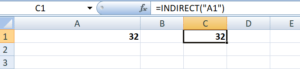In the above example, the INDIRECT function converted a text string into a cell reference. The INDIRECT function helps us put the address of one cell (A1 in our example) into another as a usual text string, and then get the value of the first cell by acknowledging the second.

### How is the INDIRECT function useful to Excel users?

Yes, it is indeed a useful function. Let’s take a few examples to understand the merits of the INDIRECT function.

For an array of numbers: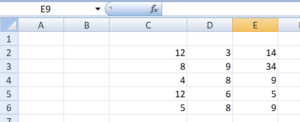When I give the formula Indirect(“D”&4), I will get 8. This is so as it will refer to D4.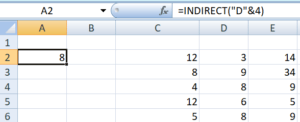When I use the formula INDIRECT(“E” & ROW() ), I used the EXCEL ROW function to return the reference to the current row number (i.e., 3), and used this to form part of the cell reference. Therefore, the Indirect formula returns the value from cell E3.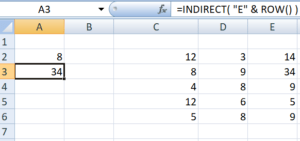When I use the formula SUM( INDIRECT( “C4:E4” ) ), the Indirect function returns a reference to the range C4:E4, and then passes this to Excel’s SUM function. The SUM function, therefore, returns the sum of cells C4, D4, and E4, that is (4 + 8 + 9).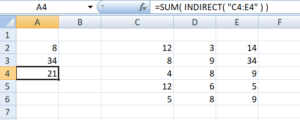Similarly, when I use the formula AVERAGE ( INDIRECT( “C5:E5” ) ), the INDIRECT function returns a reference to the range C5:E5, and then passes this to Excel’s AVERAGE function.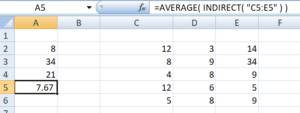The usefulness of Excel’s INDIRECT function is not just limited to building “dynamic” cell references. In addition, it can also be used to refer to cells in other worksheets.

### How to lock a cell reference using INDIRECT function

Generally, when we add or delete rows or columns in Excel, the cell references change automatically. If you wish to prevent this from happening, the INDIRECT function is quite useful.

In the screenshot below, I used the INDIRECT function for A2 and gave a plain reference for A3.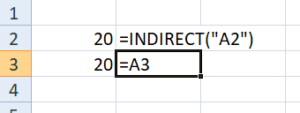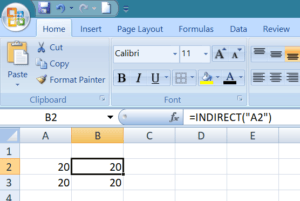When I insert a new column, what will happen? The cell equal to the logical operator still returns 20, as its formula was automatically changed to =B3.

However, the cell with the INDIRECT formula now returns 0, because the formula was not changed when a new row was inserted and it still refers to cell A1, which is currently empty: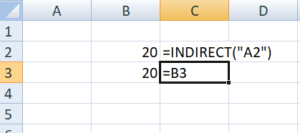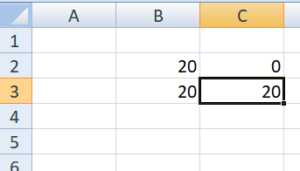So the Excel INDIRECT function would be useful in a scenario when we don’t want the formula to get changed automatically.

### Free Excel Course

To keep learning about Excel functions and developing your skills, check out our Free Excel Crash Course!  Learn how to create more sophisticated financial analysis and modeling to become a successful financial analyst.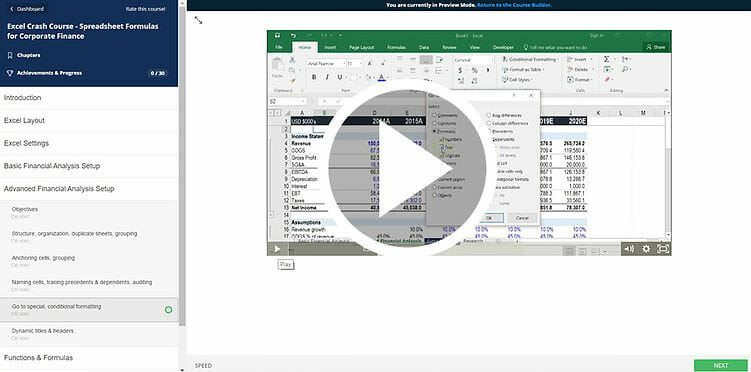Thanks for reading CFI’s guide to the important Excel INDIRECT function! By taking the time to learn and master these functions, you’ll significantly speed up your financial analysis. To learn more, check out these additional CFI resources:

• Excel Functions for Finance